【GiantPandaCV导语】这篇文章从多个角度探索了ONNX，从ONNX的导出到ONNX和Caffe的对比，以及使用ONNX遭遇的困难以及一些解决办法，另外还介绍了ONNXRuntime以及如何基于ONNXRuntime来调试ONNX模型等，后续也会继续结合ONNX做一些探索性工作。

# 0x1. 导出ONNX¶

import torch
device = torch.device("cuda" if torch.cuda.is_available() else "cpu")

model = torch.load("test.pth") # pytorch模型加载
batch_size = 1  #批处理大小
input_shape = (3, 244, 224)   #输入数据,改成自己的输入shape

# #set the model to inference mode
model.eval()

x = torch.randn(batch_size, *input_shape)   # 生成张量
x = x.to(device)
export_onnx_file = "test.onnx"      # 目的ONNX文件名
torch.onnx.export(model
x,
export_onnx_file,
opset_version=10,
do_constant_folding=True,   # 是否执行常量折叠优化
input_names=["input"],  # 输入名
output_names=["output"],    # 输出名
dynamic_axes={"input":{0:"batch_size"},  # 批处理变量
"output":{0:"batch_size"}})


• 自定义OP问题

class Focus(nn.Module):
# Focus wh information into c-space
def __init__(self, c1, c2, k=1, s=1, p=None, g=1, act=True):  # ch_in, ch_out, kernel, stride, padding, groups
super(Focus, self).__init__()
self.conv = Conv(c1 * 4, c2, k, s, p, g, act)
# self.contract = Contract(gain=2)

def forward(self, x):  # x(b,c,w,h) -> y(b,4c,w/2,h/2)
return self.conv(torch.cat([x[..., ::2, ::2], x[..., 1::2, ::2], x[..., ::2, 1::2], x[..., 1::2, 1::2]], 1))
# return self.conv(self.contract(x))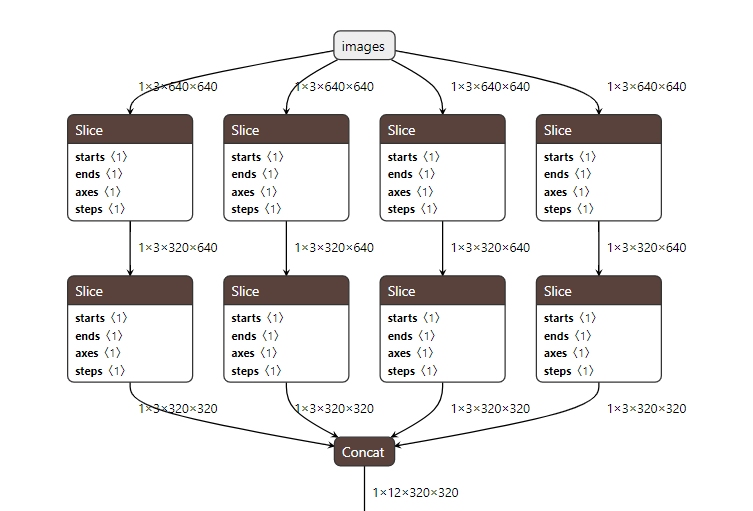• 后处理问题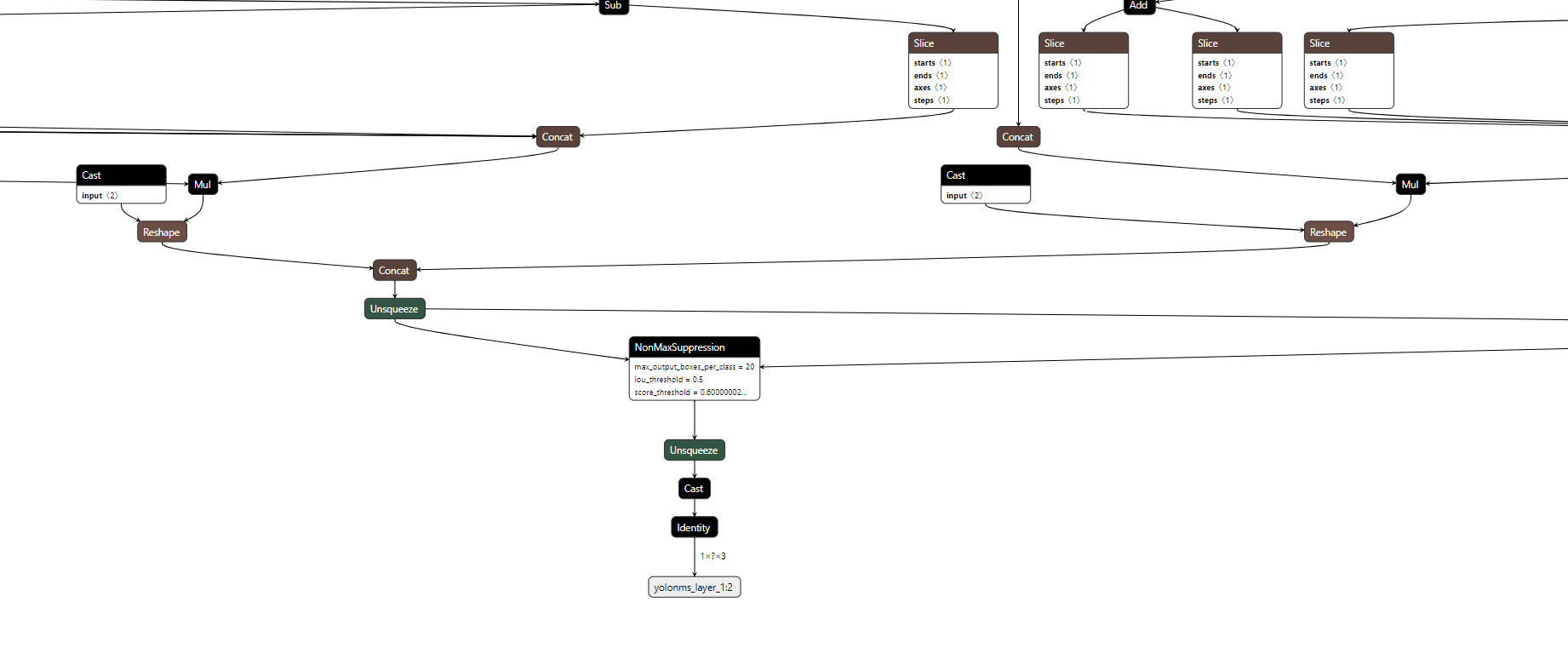• 胶水OP问题

import torch

class JustReshape(torch.nn.Module):
def __init__(self):
super(JustReshape, self).__init__()

def forward(self, x):
return x.view((x.shape, x.shape, x.shape, x.shape))

net = JustReshape()
model_name = '../model/just_reshape.onnx'
dummy_input = torch.randn(2, 3, 4, 5)
torch.onnx.export(net, dummy_input, model_name, input_names=['input'], output_names=['output'])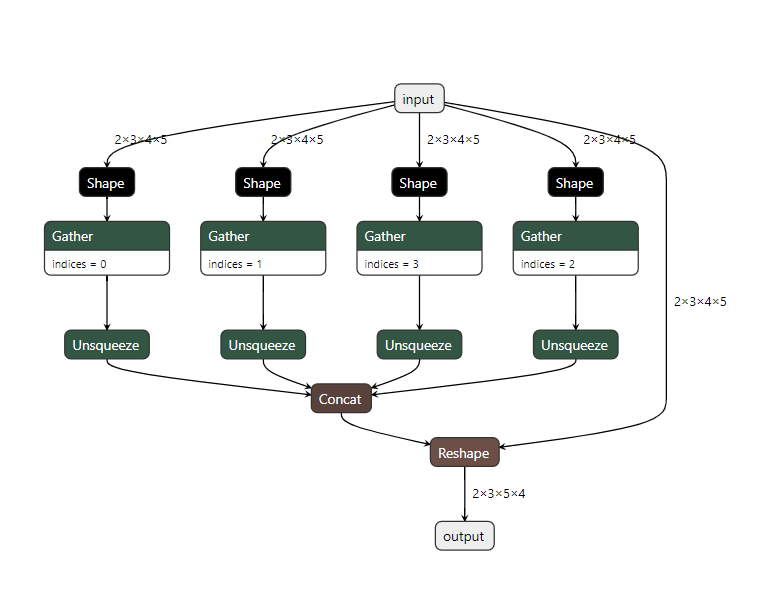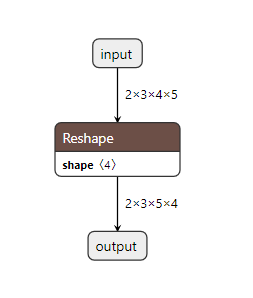# 0x2. ONNX or Caffe？¶

result = check()
if result == 0:
result = algorithm1(result)
else:
result = algorithm2(result)
return result


# 0x3. 一些典型坑点及解决办法¶

• 预处理问题

import torch

class JustReshape(torch.nn.Module):
def __init__(self):
super(JustReshape, self).__init__()
self.mean = torch.randn(2, 3, 4, 5)
self.std = torch.randn(2, 3, 4, 5)

def forward(self, x):
x = (x - self.mean) / self.std
return x.view((x.shape, x.shape, x.shape, x.shape))

net = JustReshape()
model_name = '../model/just_reshape.onnx'
dummy_input = torch.randn(2, 3, 4, 5)
torch.onnx.export(net, dummy_input, model_name, input_names=['input'], output_names=['output'])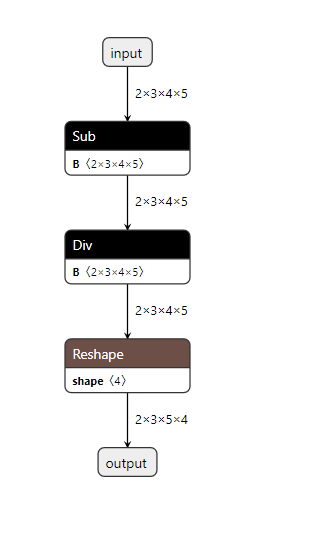• 框架OP实现不一致问题

2）[ONNXRuntimeError] : 6 : RUNTIME_EXCEPTION : Non-zero status code returned while running PRelu node. Name:'conv_1_relu'...... Attempting to broadcast an axis by a dimension other than 1. 56 by 64


graph.input.remove(input_map[input_name])
new_nv = helper.make_tensor_value_info(input_name, TensorProto.FLOAT, [input_dim_val,1,1])
graph.input.extend([new_nv])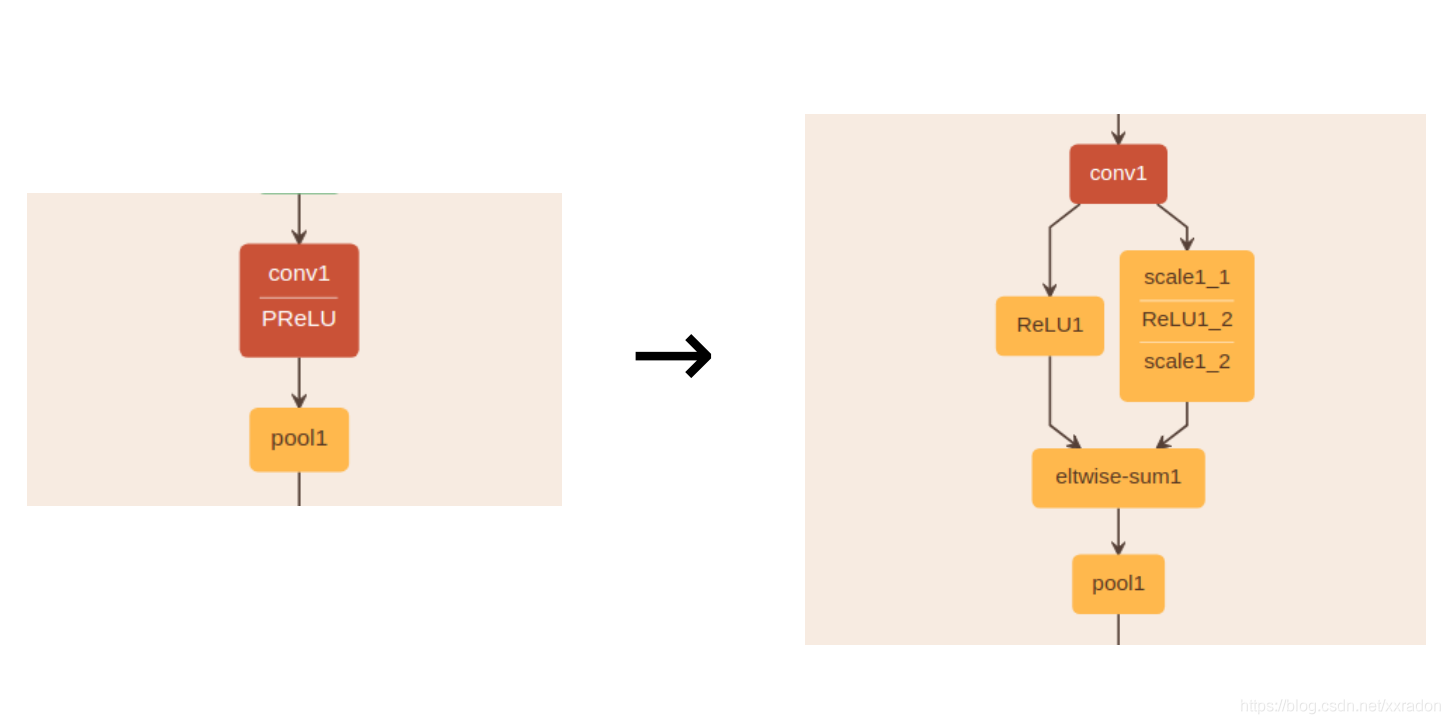• 其它问题

# 为onnx模型增加batch维度
def set_model_input_batch(self, index=0, name=None, batch_size=4):
model_input = None
if name is not None:
for ipt in self.model.graph.input:
if ipt.name == name:
model_input = ipt
else:
model_input = self.model.graph.input[index]
if model_input:
tensor_dim = model_input.type.tensor_type.shape.dim
tensor_dim.ClearField("dim_param")
tensor_dim.dim_value = batch_size
else:
print('get model input failed, check index or name')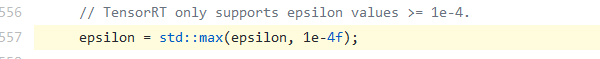# 给ONNX模型中的目标节点设置指定属性
# 调用方式为：set_node_attribute(in_node, "epsilon", 1e-5)
# 其中in_node就是所有的instancenorm op。
def set_node_attribute(self, target_node, attr_name, attr_value):
flag = False
for attr in target_node.attribute:
if (attr.name == attr_name):
if attr.type == 1:
attr.f = attr_value
elif attr.type == 2:
attr.i = attr_value
elif attr.type == 3:
attr.s = attr_value
elif attr.type == 4:
attr.t = attr_value
elif attr.type == 5:
attr.g = attr_value
# NOTE: For repeated composite types, we should use something like
# del attr.xxx[:]
# attr.xxx.extend([n1, n2, n3])
elif attr.type == 6:
attr.floats[:] = attr_value
elif attr.type == 7:
attr.ints[:] = attr_value
elif attr.type == 8:
attr.strings[:] = attr_value
else:
print("unsupported attribute data type with attribute name")
return False
flag = True

if not flag:
# attribute not in original node
print("Warning: you are appending a new attribute to the node!")
target_node.attribute.append(helper.make_attribute(attr_name, attr_value))
flag = True
return flag


# 通过op的类型获取onnx模型的计算节点
def get_nodes_by_optype(self, typename):
nodes = []
for node in self.model.graph.node:
if node.op_type == typename:
nodes.append(node)
return nodes
# 移除ONNX模型中的目标节点
def remove_node(self, target_node):
'''
删除只有一个输入和输出的节点
'''
node_input = target_node.input
node_output = target_node.output
# 将后继节点的输入设置为目标节点的前置节点
for node in self.model.graph.node:
for i, n in enumerate(node.input):
if n == node_output:
node.input[i] = node_input

target_names = set(target_node.input) & set([weight.name for weight in self.model.graph.initializer])
self.remove_weights(target_names)
self.remove_inputs(target_names)
self.remove_value_infos(target_names)
self.model.graph.node.remove(target_node)


# 0x4. ONNXRuntime介绍及用法¶

ONNXRuntime是微软推出的一个推理框架，似乎最新版都支持训练功能了，用户可以非常方便的运行ONNX模型。ONNXRuntime支持多种运行后端包括CPU，GPU，TensorRT，DML等。ONNXRuntime是专为ONNX打造的框架，虽然我们大多数人把ONNX只是当成工具人，但微软可不这样想，ONNX统一所有框架的IR表示应该是终极理想吧。从使用者的角度我们简单分析一下ONNXRuntime即可。

import numpy as np
import onnx
import onnxruntime as ort

image = np.expand_dims(image, axis=0)

sess = ort.InferenceSession(onnx_model.SerializeToString())
sess.set_providers(['CPUExecutionProvider'])
input_name = sess.get_inputs().name
output_name = sess.get_outputs().name

output = sess.run([output_name], {input_name : image_data})
prob = np.squeeze(output)
print("predicting label:", np.argmax(prob))


# 0x5. 调试工具¶

# 通过名字获取onnx模型中的计算节点
def get_node_by_name(self, name):
for node in self.model.graph.node:
if node.name == name:
return node


 # 将target_node添加到ONNX模型中作为输出节点
def add_extra_output(self, target_node, output_name):
target_output = target_node.output
extra_shape = []
for vi in self.model.graph.value_info:
if vi.name == target_output:
extra_elem_type = vi.type.tensor_type.elem_type
for s in vi.type.tensor_type.shape.dim:
extra_shape.append(s.dim_value)
extra_output = helper.make_tensor_value_info(
output_name,
extra_elem_type,
extra_shape
)
identity_node = helper.make_node('Identity', inputs=[target_output], outputs=[output_name], name=output_name)
self.model.graph.node.append(identity_node)
self.model.graph.output.append(extra_output)


def show_node_attributes(node):
print("="*10, "attributes of node: ", node.name, "="*10)
for attr in node.attribute:
print(attr.name)
print("="*60)


def show_node_inputs(node):
# Generally, the first input is the truely input
# and the rest input is weight initializer
print("="*10, "inputs of node: ", node.name, "="*10)
for input_name in node.input:
print(input_name)  # type of input_name is str
print("="*60)


...

# 0x7. 参考资料¶